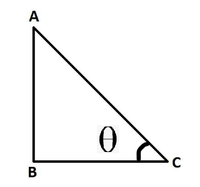Open in App
Not now

# Prove that tan4θ + tan2θ = sec4θ – sec2θ

• Last Updated : 17 Nov, 2022

Trigonometry is the branch of mathematics that deals with triangles and angles associated with them. If the word is broken into 2 parts namely trigon and geometry, It can be understood easily that this branch of mathematics deals with the geometry of triangles. Using trigonometry, the properties of triangles and their applications can be easily understood. Using trigonometry, one can find the angles and missing sides of any triangle with the use of trigonometric ratios.

### Trigonometric Ratios

There are six functions or trigonometric ratios of an angle that are present in trigonometry. Their names and abbreviations are sine (sin), cosine (cos), tangent (tan), cotangent (cot), secant (sec), and cosecant (csc). One important thing to note here is that trigonometric formulas work only on the right-angle triangle. Let’s see the below image.In this right-angle triangle, side AC is known as hypotenuse. side BC is known as the base of the triangle. side AB is known as the height of the triangle.

Basic formulas of trigonometric ratios

• Tan ∅ = sin ∅/cos ∅
• sin ∅ = 1/cosec ∅
• cos ∅= 1/sec ∅
• Tan ∅= 1/cot ∅

### Prove that tan4θ + tan2θ = sec4θ – sec2θ

Before starting to solve the below proof, it is important to know some basic identities that are associated with trigonometry. These formulas must be by-hearted in order to solve problems related to trigonometry. below are the formulas,

tan2∅ + 1 = sec2

In the above problem, LHS is tan4θ + tan2θ

Take tan2 θ common from above

i.e, tan2θ(tan2θ + 1)  ⇢  (i)

From the formula, sec2∅+1=tan2∅, substituting this in (i),

tan2∅(sec2∅)  ⇢  (ii)

Now, the RHS is sec4 θ – sec2 θ, so transform the tan2∅  present in (ii) to sec2∅ form.

So, using the same formula tan2∅ + 1 = sec2∅, we can also write this as  tan2∅=sec2∅-1

Substituting this in (ii),

(sec2∅ – 1)(sec2∅)

= sec4∅  -sec2

= RHS

Hence proved

### Similar Problems

Question 1: Prove that sec2θ + cosec2θ = sec2θ × cosec2θ

Solution:

It is known, cos∅ = 1/sec ∅ or sec∅ = 1/cos∅

And sin∅ = 1/cosec∅ or cosec∅ = 1/sin ∅

Substituting these formula in LHS,

1/cos2∅  + 1/sin2∅

Taking LCM,

(sin2∅ + cos2∅)/sin2∅ cos2∅

sin2∅ + cos2∅ = 1, therefore,

LHS = 1/sin2∅ cos2

Now coming to RHS,

cos∅ = 1/sec∅ or sec∅ = 1/cos∅

And sin  = 1/cosec∅ or cosec∅ = 1/sin∅,

So substituting these formulas in RHS,

1/sin2∅ cos2∅ = RHS = LHS

Hence proved.

Question 2: Prove that (sin∅ + cosec∅)2 + (cos∅ + sec∅)2 = 7 + tan2∅ + cot2

Solution:

It is known, (a + b)2 = a2 + b2 + 2ab,

Applying this formula to LHS,

sin2∅ + cosec2∅ + 2sin∅ cosec∅ + cos2∅ + sec2∅ + 2cos∅ sec∅

• sin ∅ = 1/cosec ∅
• cos ∅ = 1/sec ∅

Putting these in the above equation,

sin2∅ + cosec2∅ + 2sin∅ 1/sin ∅ + cos2∅ + sec2∅ + 2cos∅ 1/cos ∅

= sin2∅ + cosec2∅ +2 + cos2∅ + sec2∅ + 2

= (sin2∅ + cos2∅) + cosec2∅ + sec2∅ + 4

= 1+ cosec2∅ + sec2∅ + 4

= (1+ tan2θ) + (1+ cot2θ) + 5              [sec2θ = 1 + tan2θ;  cosec2θ = 1+ cot2θ ]

= 7 + tan2∅ + cot2

Hence proved

My Personal Notes arrow_drop_up